Seepage Analysis

# Seepage Analysis - Civil Engineering SSC JE (Technical) - Civil Engineering (CE)

Chapter 6 Seepage Analysis

SEEPAGE PRESSURE AND SEEPAGE FORCE
Seepage pressure is exerted by the water on the soil due to friction drag. This drag force/seepage force always acts in the direction of flow.
The seepage pressure is given by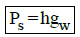where,Ps = Seepage Pressure gw = 9.18 km/m3.

here, h = head loss and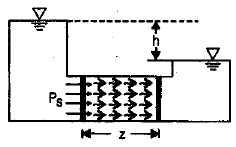(ii) Fs =hAgw

where, Fs = Seepage force.

(iii) fs =igw
where , fs = Seepage force per unit volume.
i =h / z
NOTE: Ps, Fs and fs acts in the same direction i.e, in the direction of flow.

EFFECTIVE STRESSES: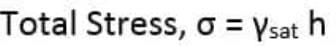-The pore water pressure (u) is the pressure caused due to the water molecules present in the pores or voids of the soil. It is given by the relation: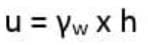Where,γw=Unit weight of water
Pore water pressure can also be designated as neutral pressure or neutral stress because of the fact that it cannot resist the shear forces in the soil mass.
Note: When the pressure is equal to atmospheric pressure, the pore water pressure is taken as zero.

-The effective stress is given by the formula: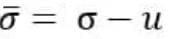For saturated soil, Effective stress is given by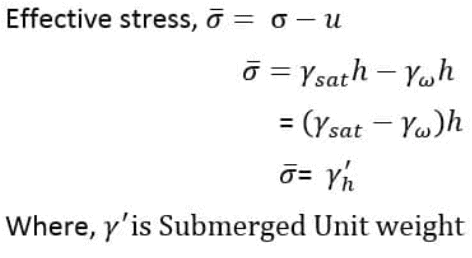QUICK SAND CONDITION
Quicksand condition is the floatation of particles of cohesionless soil, like fine gravel and sand, due to vertical upward seepage flow. As sand boiling occurs, the bearing capacity and shear strength of the cohesionless soil decrease and the agitations of soil particles become apparent.
How Quicksand Condition Occurs?
Quicksand condition occurs when seepage pressure, which acts in the upward direction, overcomes the downward direction pressure due to submerged weight of soil, and the sand grains are forced apart. The result is that the soil has no capability to support a load.

The soil that experiences quicksand condition would lose shear strength and bearing capacity. The shear strength of cohesionless soil depends on the effective stress. The shear strength is given by: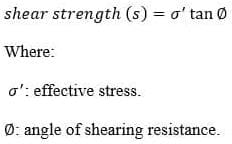The effective stress is given by the following expression: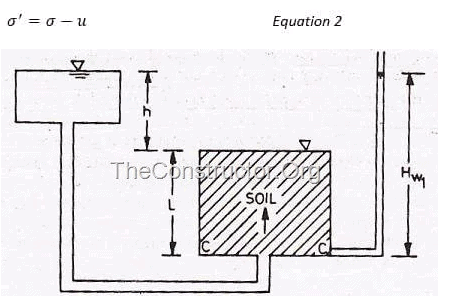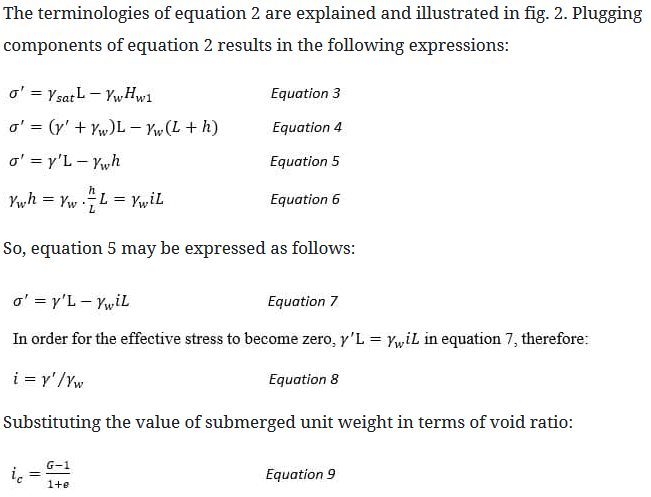Taking G=2.67, and e=0.67, the result of equation 9 is equal to one.

Thus, the effective stress becomes zero for the soil with above values of G and e, when the hydraulic gradient ‘i’ is unity, i.e. head causing the flow is equal to the length of the specimen.

LAPLACE EQUATION OF TWO DIMENSHIONAL FLOW AND FLOW NET:

(i)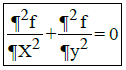where, f = hydraulic head or head difference between upstream and downstream level or head loss through the soil.

(ii)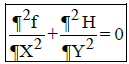...2D Laplace equation for
Homogeneous soil. where, f = kx H and f = ky . H for lsotropic soil,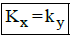SEEPAGE DISCHARGE (q)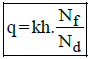where, h = hydraulic head or head difference between upstream and downstream level or head loss through the soil.

 shapefactor =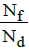where,Nf = Total number of flow channels N1/2 = Total number of flow lines.

N= Ny - 1
where, Nd = Total number equipotential drops.
Nf = Total number equipotential lines.

Hydrostatic pressure = U = hwgw
where, U = Pore pressure

• Seepage Pressure

Ps =h 'gw
where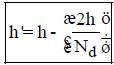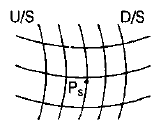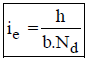where, Size of exit flow field is b × b.
and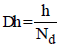is equipotential drop.

PHREATIC LINE
It is top flow line which follows the path of base parabola. It is a stream line. The pressure on this line is atmospheric (zero) and below this line pressure is hydrostatic.

(a) Phreatic Line with Filter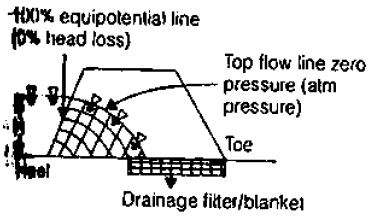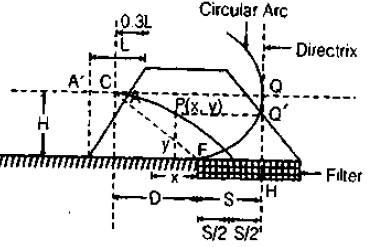Phreatic line ( Top flow line). ¯
Flows the path of base parabola
CF = Radius of circular arc =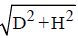C = Entry point of base  parabola

F = Junction of permeable and impermeable   surface
S = Distance between focus and directrix = Focal length
FH = S

(i) q = ks
where, q = Discharge through unit length of dam.
(ii)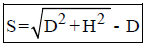(iii)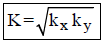® For 2D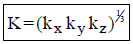® For 3D

(b) Phreatic Line without Filter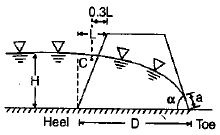(i) For a< 30º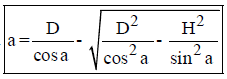(ii) For a> 30º

 q = k a sin a tan a

and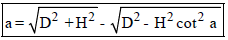For neglecting the flow of sand of down stream. Some Filte is used.
Remember:-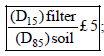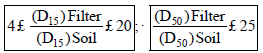The document Seepage Analysis | Civil Engineering SSC JE (Technical) - Civil Engineering (CE) is a part of the Civil Engineering (CE) Course Civil Engineering SSC JE (Technical).
All you need of Civil Engineering (CE) at this link: Civil Engineering (CE)

## Civil Engineering SSC JE (Technical)

2 videos|122 docs|55 tests

## FAQs on Seepage Analysis - Civil Engineering SSC JE (Technical) - Civil Engineering (CE)

 1. What is seepage analysis in civil engineering?Ans. Seepage analysis in civil engineering refers to the study and evaluation of the flow of water through porous materials, such as soil or concrete. It involves analyzing the behavior of water under different conditions, such as pressure gradients, hydraulic conductivity, and soil properties, to assess the potential risks of seepage and its impact on structures.
 2. How is seepage analysis conducted in civil engineering?Ans. Seepage analysis in civil engineering is typically conducted using numerical methods, such as finite element analysis or finite difference method. These methods involve dividing the region of interest into smaller elements, applying governing equations for fluid flow, and solving the equations iteratively to obtain the seepage characteristics and potential flow paths.
 3. What are the factors considered in seepage analysis?Ans. Several factors are considered in seepage analysis in civil engineering, including hydraulic conductivity of the soil or material, hydraulic gradient, boundary conditions, porosity, and permeability. Additionally, factors like the presence of cracks, joints, or other paths for water flow are also considered to assess the potential for seepage.
 4. Why is seepage analysis important in civil engineering projects?Ans. Seepage analysis is crucial in civil engineering projects as it helps in understanding and predicting the behavior of water flow through porous materials. It allows engineers to assess the risks associated with seepage, such as soil erosion, stability of structures, potential for foundation failure, and impact on nearby structures or the environment. This analysis helps in designing effective drainage systems and implementing appropriate measures to mitigate seepage-related issues.
 5. What are the common methods to mitigate seepage in civil engineering?Ans. There are several common methods to mitigate seepage in civil engineering, including the use of impermeable liners or membranes, grouting or injection of chemical substances to seal cracks or voids, installation of drainage systems such as weep holes or French drains, and slope stabilization techniques. These methods are implemented based on the specific conditions and requirements of the project to effectively reduce or prevent seepage.

## Civil Engineering SSC JE (Technical)

2 videos|122 docs|55 testsExplore Courses for Civil Engineering (CE) examSignup to see your scores go up within 7 days! Learn & Practice with 1000+ FREE Notes, Videos & Tests.
10M+ students study on EduRev
Track your progress, build streaks, highlight & save important lessons and more!
Related Searches

,

,

,

,

,

,

,

,

,

,

,

,

,

,

,

,

,

,

,

,

,

;